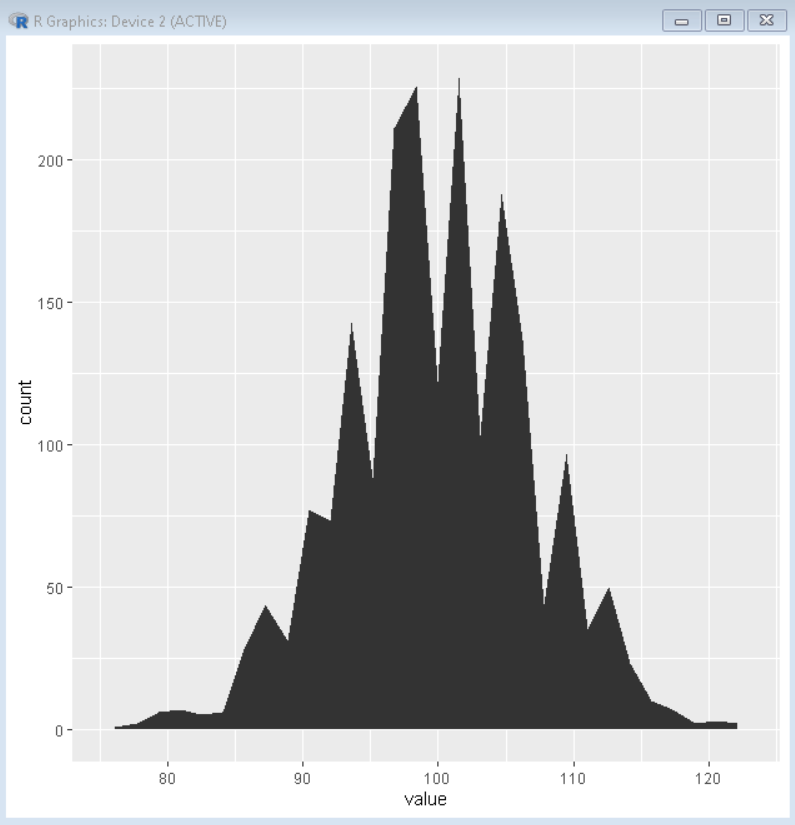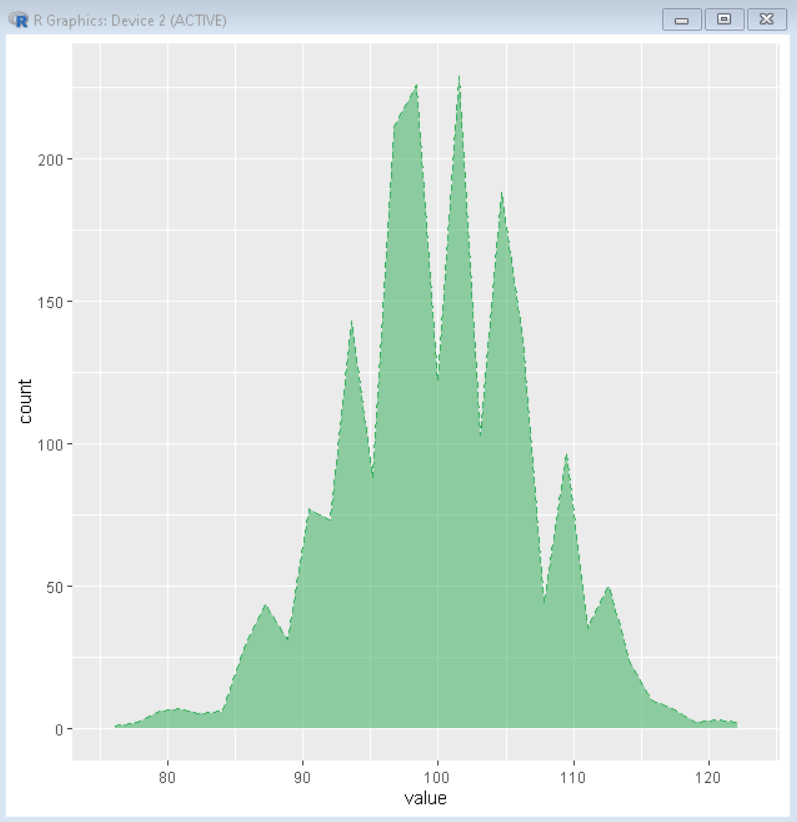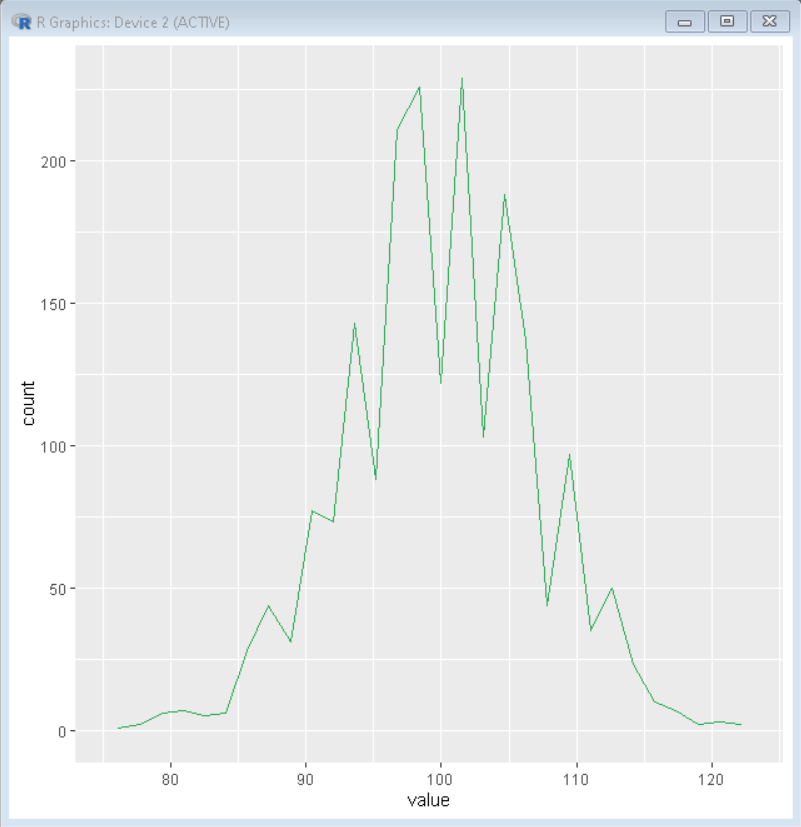GeeksforGeeks App
Open AppBrowser
Continue

# geom_area plot with areas and outlines in ggplot2 in R

An Area Plot helps us to visualize the variation in quantitative quantity with respect to some other quantity. It is simply a line chart where the area under the plot is colored/shaded. It is best used to study the trends of variation over a period of time, where we want to analyze the value of one variable change over a period of time or respect to any other variable.

In this article, we will discuss how to draw an area plot in the R Programming Language using the ggplot2 package. To do so we use the geom_area() function that helps us create the area plot layer.

Syntax: geom_area(mapping, data , stat , position)

Argument:

• mapping: determines the aesthetic mapping usually constructed with aes() function.
• data: determines the data frame to be used for mapping.
• stat: determines the statistical transformation.
• position: determines the position adjustment for overlapping points.

Example:

Here is a basic area plot using the geom_area() function.

## R

 `# create dataframe``set.seed``(1234)``df <- ``data.frame``(value =``round``(``c``(``rnorm``(2000,``                                      ``mean=100,``                                      ``sd=7))))`` ` `# import libraries ggplot2``library``(ggplot2)  `` ` `# create area plot`` ``ggplot``(df, ``aes``(x=value)) + ``geom_area``(stat = ``"bin"``)`

Output:## Color and Linetype Customization

We can customize the color of the plot fill, outline as well as line type of outline using the color, fill, and linetype parameters of the geom_area() function.

Syntax: plot + geom_area( color, fill, linetype, alpha)

Parameters:

• color: determines the color of the outline of the area plot.
• fill:  determines the color of background fill.
• linetype: determines the type of outline in the plot.
• alpha: determines the transparency of plot fill.

Example:

Here is an area plot with green color fill and a green outline with a dashed line and 50% transparency.

## R

 `# create dataframe``set.seed``(1234)``df <- ``data.frame``(value =``round``(``c``(``rnorm``(2000,``                                      ``mean=100,``                                      ``sd=7))))`` ` `# import libraries ggplot2``library``(ggplot2)  `` ` `# create area plot``# color, fill and linetype parameters``# are used for color customization`` ``ggplot``(df, ``aes``(x=value)) + ``            ``geom_area``(stat = ``"bin"``, color = ``"#2bab53"``, ``                      ``fill = ``"#2bab53"``, linetype = ``"dashed"``, ``                      ``alpha = 0.5)`

Output:## Outline Plot

To create an outline plot using the geom_area() function, we create a basic area plot with transparency set to zero percent using the alpha parameter of the geom_area() function.

Syntax: geom_area( alpha=0 )

Example:

Here, is an outline plot with a green outline made using the geom_area() function.

## R

 `# create dataframe``set.seed``(1234)``df <- ``data.frame``(value =``round``(``c``(``rnorm``(2000,``                                      ``mean=100,``                                      ``sd=7))))`` ` `# import libraries ggplot2``library``(ggplot2)  `` ` `# create area plot``# alpha as zero is used for converting area plot to line plot`` ``ggplot``(df, ``aes``(x=value)) + ``            ``geom_area``(stat = ``"bin"``, color = ``"#2bab53"``,``                      ``alpha = 0)`

Output:My Personal Notes arrow_drop_up